# Nature of Roots

Nature of Roots

In this mini-lesson, we will explore about the nature of roots of a quadratic equation.

You will learn about the nature of roots of quadratic equation using the discriminant formula, quadratic formula, roots of a cubic equation, real roots, unreal roots, irrational roots, imaginary roots and other interesting facts around the topic. You can also check out the playful calculators to know more about the lesson and try your hand at solving a few interesting practice questions at the end of the page.

The word "Quadratic" is derived from the word "Quad" which means square.

In other words, a quadratic equation is an “equation of degree 2.”

There are many scenarios where quadratic equations are used.

Did you know that when a rocket is launched, its path is described by a quadratic equation?

## Lesson Plan

 1 What Do You Mean By Nature of Roots? 2 Important Notes on Nature of Roots 3 Solved Examples on Nature of Roots 4 Challenging Question on Nature of Roots 5 Interactive Questions on Nature of Roots

## What Do You Mean By Nature of Roots?

An equation of the form $$ax^2+bx+c=0$$, where $$a \neq 0$$ is called a quadratic equation.

The standard form of a quadratic equation looks like this: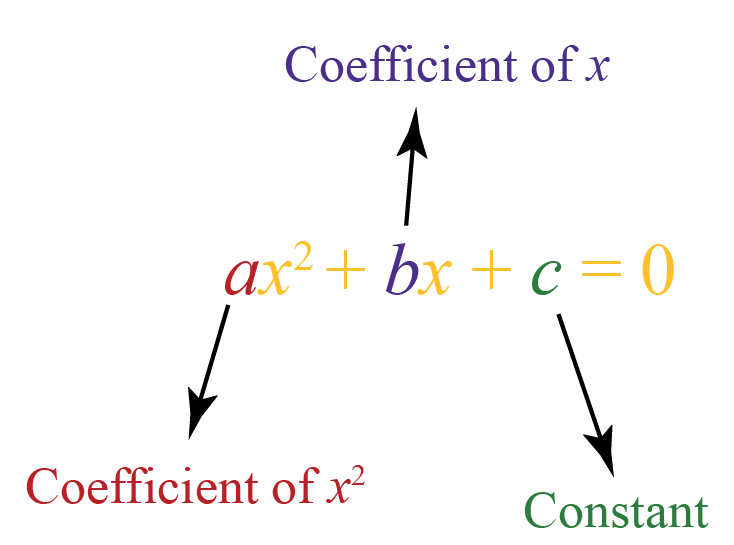This is a quadratic equation in variable $$x$$.

But always remember that $$a$$ is a non-zero value.

Now, to find the nature of the roots of the quadratic equation, we will first calculate the roots of the equation.

Nature of roots specifies that the equation has real roots, irrational roots, or imaginary roots.

Imaginary roots are also known as unreal roots.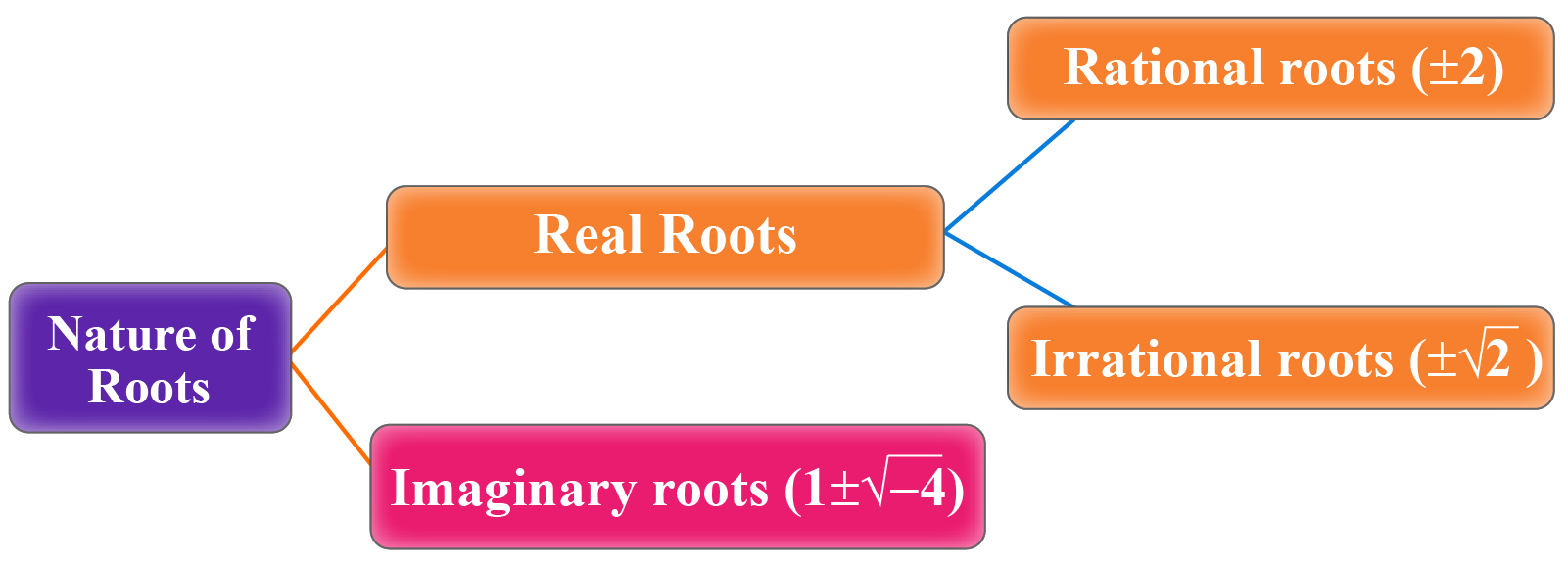## How Do You Find the Nature of the Roots?

Here is the quadratic formula used to find the roots of a quadratic equation.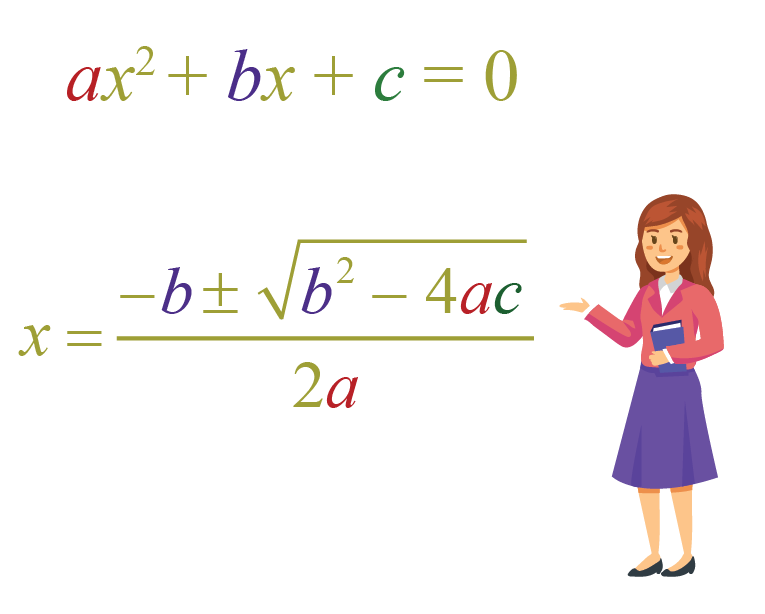### Discriminant Formula

Do you observe the term $$b^2-4ac$$ in the quadratic formula?

This is called a "discriminant" because it discriminates the roots of the quadratic equation based on its sign.

The discriminant is used to find the nature of roots of a quadratic equation.

• $$b^2-4ac>0$$  -  In this case, the quadratic equation has two distinct real roots.
• $$b^2-4ac=0$$  -  In this case, the quadratic equation has one repeated real root.
• $$b^2-4ac<0$$  -  In this case, the quadratic equation has no real root.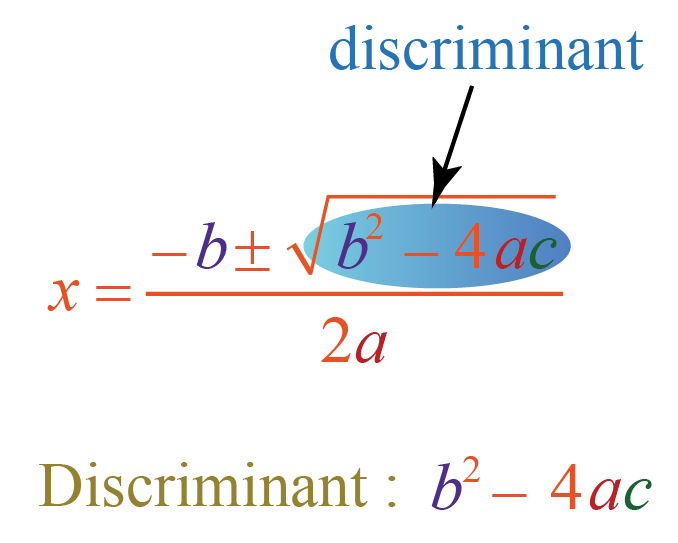### Example

Let us consider the quadratic equation $$2x^2+x-3=0$$

Let’s find the determinant to find the nature of the roots.

\begin{align}b^2-4ac&=1^2-4\times 2\times (-3)\\&=1+24\\&=25>0\end{align}

This means that the quadratic equation has two real and distinct roots.

Look at the graph of this quadratic equation shown below.This is how the quadratic equation is represented on a graph.

This curve is called a parabola.

Observe that the parabola intersects the $$x$$-axis at two distinct points.

The zeroes of the quadratic equation $$2x^2+x-3=0$$ are the $$x$$ coordinates of the points where the graph of the polynomial intersects the $$x$$-axis, that is, $$-\dfrac{3}{2}$$ and 1

### Types of Roots

There are three types of roots of a quadratic equation $$ax^2+bx+c=0$$:

1. Real and distinct roots
2. Real and equal roots
3. Complex roots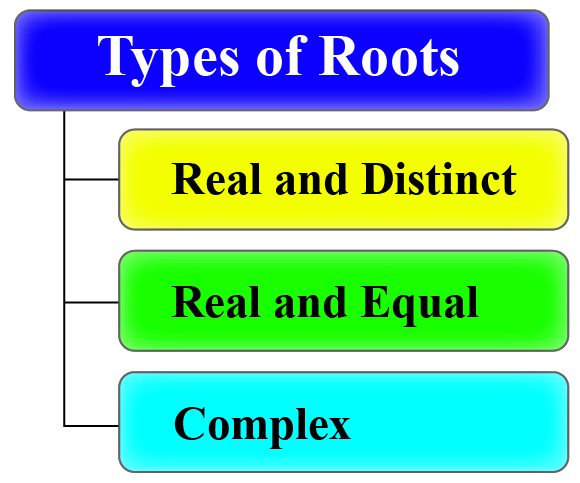### Real and Distinct Roots

• The discriminant is positive, that is, $$b^2-4ac>0$$
• The curve intersects the $$x$$-axis at two distinct points.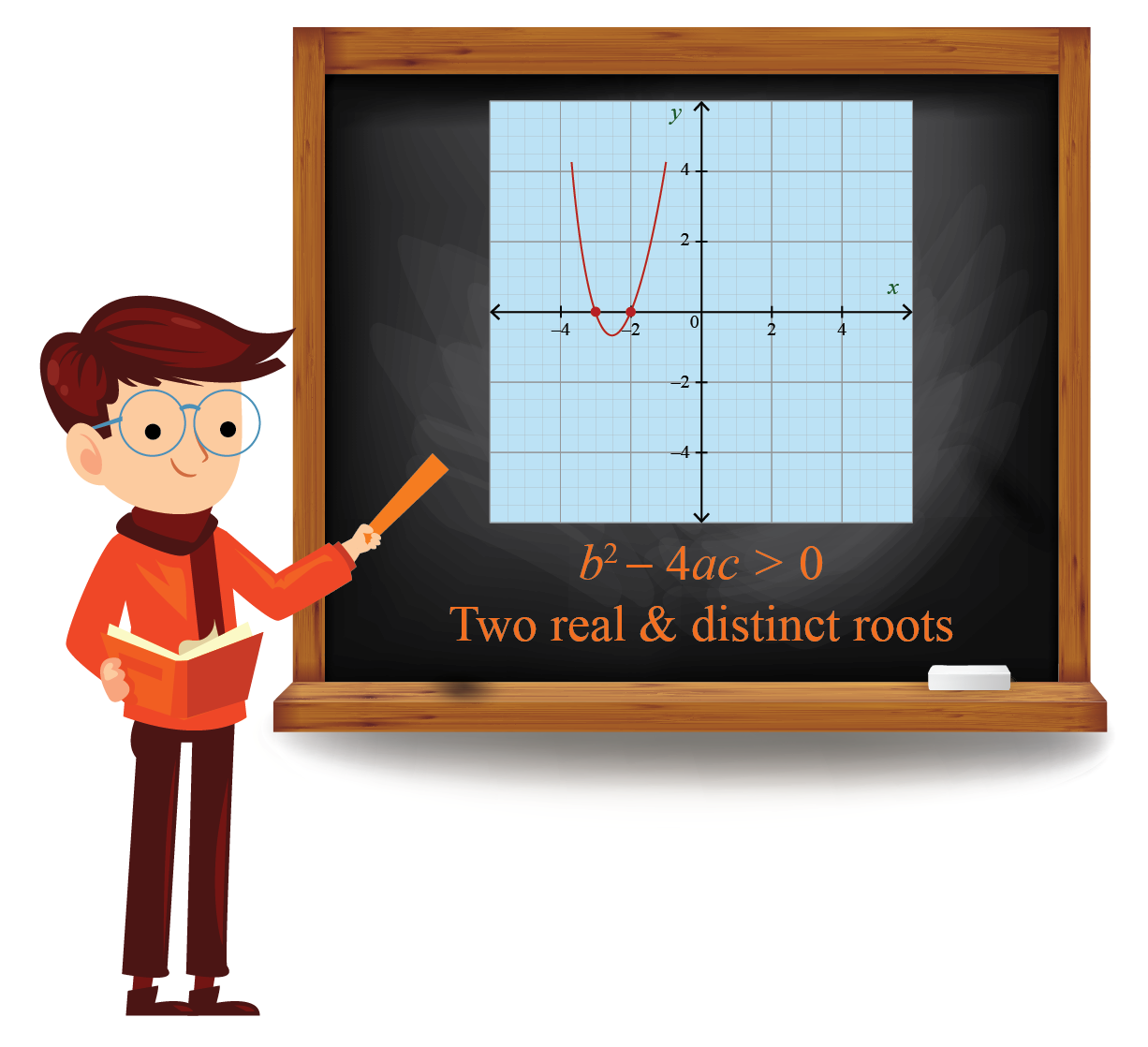### Real and Equal Roots

• The discriminant is equal to zero, that is, $$b^2-4ac=0$$
• The curve intersects the $$x$$-axis at only one point.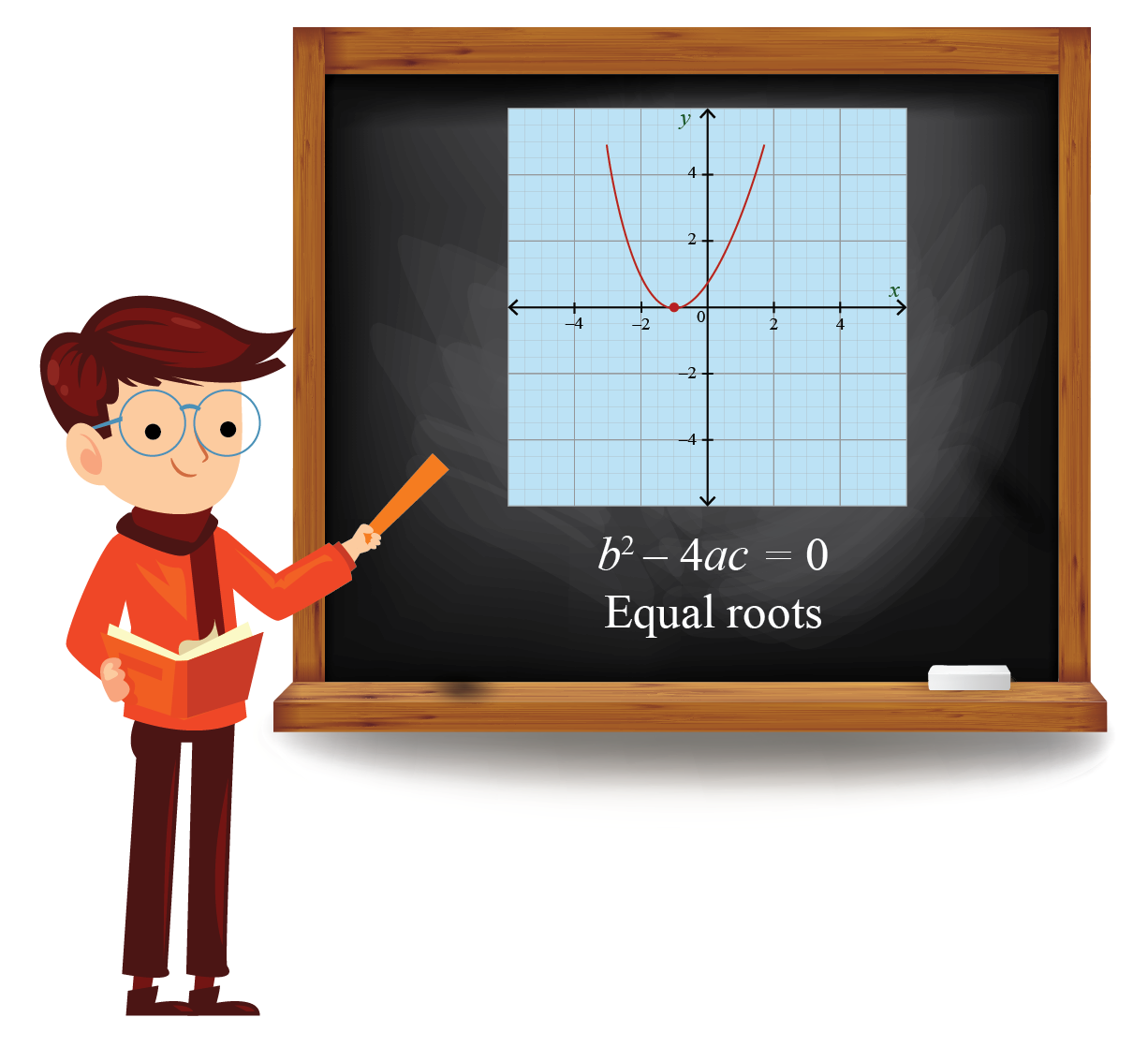### Complex Roots

• The discriminant is negative, that is, $$b^2-4ac<0$$
• The curve does not intersect the $$x$$-axis.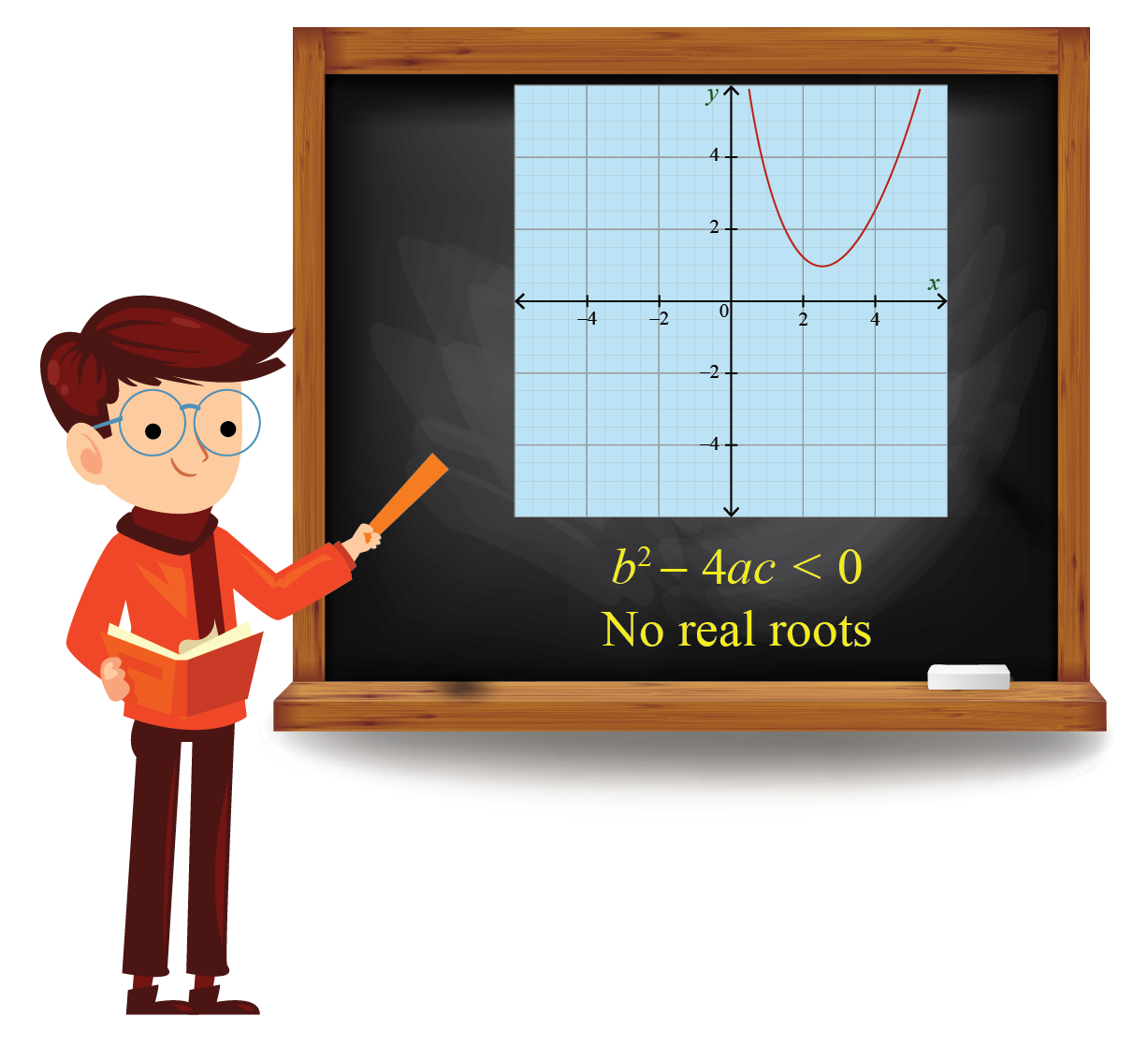### Nature of Roots Calculator

Use the below simulation to find the nature of roots of a quadratic equation graphically.

Use the sliders to adjust the values of $$a,b$$, and $$c$$, the simulation then gives the value of the discriminant, the number of real roots, and shows the graph of the quadratic equation.

## Solved Examples

 Example 1

Elsie has a two-digit secret number.

She gives a few hints to her friend Mia to crack it.She says, "It is the value of the discriminant of the quadratic equation $$x^2+9x+14=0$$."

Can you guess the lucky number?

Solution

The quadratic equation is $$x^2+9x+14=0$$

On comparing it with $$ax^2+bx+c=0$$, we get $$a=1$$, $$b=9$$, and $$c=14$$

Let's use the discriminant formula to find the discriminant.

\begin{align}\text{Discriminant}&=b^2-4ac\\&=9^{2}-4(1)(14)\\&=81-56\\&=25\end{align}

 $$\therefore$$ The lucky number is 25
 Example 2

Flora loves collecting flowers.She wants to choose a number $$k$$ such that the quadratic equation $$kx^{2}-2kx+6=0$$ has real and equal roots.

Each of the flowers has a number and the answer to this question is shown on the flowers.

Can you help her find the answer?

Solution

Compare the quadratic equation $$kx^{2}-2kx+6=0$$ with the standard form of the quadratic equation $$ax^{2}+bx+c=0$$.

We get $$a=k$$, $$b=-2k$$ and $$c=6$$

As both the roots of the quadratic equation are real and equal, the value of the discriminant $$b^2-4ac$$ is zero.

\begin{aligned}b^2-4ac&=0\-2k)^2-4(k)(6)&=0\\4k^2-24k&=0\\4k(k-6)&=0\\k&=0,6\end{aligned} Now the flowers do not contain the number 0 Therefore, $k=6$  \(\therefore, The correct number is 6Challenging Questions

Riley is trying to draw the graph of a quadratic equation. The conditions for the nature of the roots of a quadratic equation are given as:

• Both the roots are real
• One of the roots is negative and the other root is positive

Can you help her draw the possible sketches of the quadratic equation?

## Interactive Questions

Here are a few activities for you to practice.

## Let's Summarize

The mini-lesson targeted the fascinating concept of Nature of Roots. The math journey around Nature of Roots starts with what a student already knows, and goes on to creatively crafting a fresh concept in the young minds. Done in a way that is not only relatable and easy to grasp but will also stay with them forever. Here lies the magic with Cuemath.

At Cuemath, our team of math experts is dedicated to making learning fun for our favorite readers, the students!

Through an interactive and engaging learning-teaching-learning approach, the teachers explore all angles of a topic.

Be it worksheets, online classes, doubt sessions, or any other form of relation, it’s the logical thinking and smart learning approach that we, at Cuemath, believe in.

## FAQs on Nature of Roots

### 1. How do you find the nature of roots without solving them?

To find the nature of roots of a quadratic equation, the discriminant can be used.

The value of the discriminant tells us the nature of the roots of the quadratic equation.

### 2. What is the nature of roots when the discriminant is 0?

When the value of the discriminant of a quadratic equation $$ax^2+bx+c=0$$ is zero, the equation will have real and equal roots.

### 3. What are the three types of nature of roots?

There are three types of roots for a quadratic equation $$ax^2+bx+c=0$$:

1. Real and distinct roots
2. Real and equal roots
3. Complex roots

### 4. What is a real root?

A real root is a root of a quadratic equation $$ax^2+bx+c=0$$ which belongs to the set of real numbers.

### 5. What are imaginary roots?

The roots that are not represented on a number line are imaginary roots. Imaginary roots occur when the quadratic equation does not cut the $$x$$-axis.

### 6. How will you approximate the nature of roots that are irrational?

We can approximate the nature of roots that are irrational by rounding the number to two digits after the decimal point.

Example: The irrational root $$\pm1.4142135...$$ can be rounded as: $$\pm1.41$$

### 7. What are the sum and product of the roots?

The sum of zeroes of a quadratic polynomial $$ax^2+bx+c=0$$ is, $$\alpha+\beta=-\dfrac{b}{a}$$.

The product of zeroes of a quadratic polynomial $$ax^2+bx+c=0$$ is, $$\alpha\times\beta=\dfrac{c}{a}$$.

### 8. What is the nature of the roots of the quadratic equation?

There are three types of roots of a quadratic equation $$ax^2+bx+c=0$$:

1.     Real and distinct roots
2.     Real and equal roots
3.     Complex roots

### 9. How do you find the nature of the roots of a cubic equation?

To determine the nature of the roots of a cubic equation, calculate the value of its discriminant.

If the value of the discriminant is zero and all the coefficients of the cubic equations are real, then the cubic equation has real roots.

Therefore, the value of discriminant and the coefficients of the cubic equation helps us find the nature of roots of a cubic equation.

### 10. How to identify types of roots in quadratic equations?

To find the nature of roots of a quadratic equation, the discriminant can be used. The value of the discriminant tells us the types of roots of quadratic equations.

More Important Topics
More Important Topics